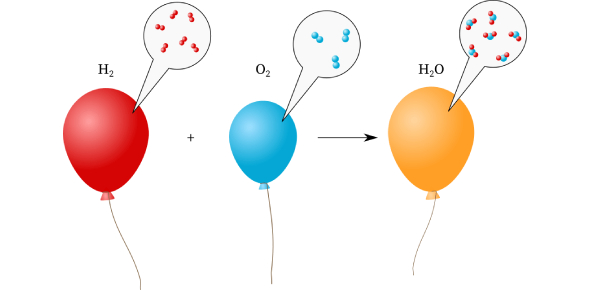# Basic Gas Chemistry Quiz

10 Questions | Total Attempts: 2214SettingsGas is a common state of matter, they are easy to compress, they expand to fill their containers and occupy more space than the liquids or solids, which they form. Take up the gas chemistry quiz below and get to know what else you have understood from the topic so far.

• 1.
Which of the following changes would INCREASE the pressure of a gas in a closed container?
• A.

Increased volume of container

• B.

Increased number of particles in the container

• C.

Decreased temperature

• 2.
Which of the following is NOT true about gases?
• A.

Gases have an indefinite volume

• B.

Gases have less kinetic energy than the same substance as a liquid.

• C.

Gases are compressible

• D.

Gases are farther apart than liquids.

• 3.
Which of the following are standard pressures:I: 1 atomosphereII 760 mm HgIII.100 mm HgIV. 100 kPaV. 101.3 kPaVI: 1 mm Hg
• A.

I, II and III are Standard Pressure for gases

• B.

I, III and V are Standard Pressure for gases

• C.

I, II and V are Standard Pressure for gases

• D.

IV and VI are Standard Pressure for gases

• 4.
What is standard temperature for gases?I. 100 degrees CelsiusII. 0 degrees CelsiusIII. 100 degrees FahrenheitIV. 0 KelvinV.   273.16 Kelvin
• A.

I (only) is correct

• B.

II (only) is correct

• C.

III (only) is correct

• D.

II and V are correct

• E.

II and IV are correct

• 5.
Where would air pressure be higher?
• A.

At the top of the Alps mountains

• B.

At sea level

• C.

• 6.
Which would have more gas pressure?
• A.

1 moles of gas in a 22.4 L container (at STP).

• B.

2 moles of gas in a 22.4 L container (at STP).

• C.

2 moles of gas in a 11.2 L container (at STP).

• 7.
Where would molecules be farther apart?
• A.

When the substance is a gas

• B.

When the substance is a liquid

• C.

When the substance is a solid

• 8.
Which of the following is NOT assumed by the kinetic-molecular theory?
• A.

Gas molecules are not significantly attracted to other gas molecules.

• B.

Collision between gas particles are elastic.

• C.

All gas molecules have the same mass.

• D.

All gas molecules move in a straight, constant motion.

• 9.
Which variable is most important to determine the average kinetic energy of gas particles?
• A.

Volume of the container

• B.

Volume of the particle

• C.

Mass of the container

• D.

Temperature

• 10.
The number of atoms in one mole of gold is:
• A.

Avagodro's number

• B.

1.02 x 10^23

• C.

Dalton's number

• D.

Boyle's number

Related Topics